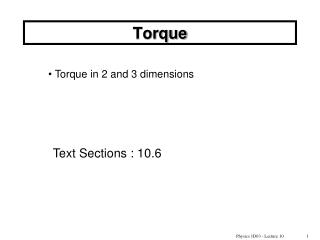DownloadDownload PresentationTorque

# Torque

Télécharger la présentation## Torque

- - - - - - - - - - - - - - - - - - - - - - - - - - - E N D - - - - - - - - - - - - - - - - - - - - - - - - - - -
##### Presentation Transcript

1. Torque • Torque in 2 and 3 dimensions Text Sections : 10.6 Physics 1D03 - Lecture 10

2. F a F -F Torque is what causes rotation An unbalanced force applied to an object causes it to move (accelerate). Even with no net force, the object is not necessarily in equilibrium. A net torque will cause it to rotate. Physics 1D03 - Lecture 10

3. A force directed toward the axis of rotation produces no torque. You cannot shut a door by pushing directly toward the hinges (F1), or pulling directly away from the hinges (F2). hinge F1 F2 Physics 1D03 - Lecture 10

4. Divide the force vector into radial and tangential components. Only the tangential component produces a torque about the rotation axis. Torque = (tangential force)  (distance to rotation axis) (is the angle between rand F) r Units: N•m This is identical to the unit of energy (joule), but is always called “N•m” when it refers to torque. Ft  Fr F Physics 1D03 - Lecture 10

5. Another way to look at torque... “effective lever arm”, r sin f The shortest distance from the pivot to the line of action of the force is the “effective lever arm”. If the force were applied at right angles to this shorter distance, the same torque would result. “line of action” of F r Torque = (force)  (effective lever arm)  F Physics 1D03 - Lecture 10

6. Torque as a vector Vector product or “cross product” of 2 vectors: B B  A Direction: C is perpendicular to A and B (out of the page, towards you). Right-hand rule: Fingers parallel to A when straight, and along B as you curl them; then the thumb gives the direction of C. Not commutative! Physics 1D03 - Lecture 10

7. The cross-product is used to define torque as a vector. t F F Φ r The direction of  is along the axis about which the body would tend to rotate. Right-hand rule: Thumb in direction of t, fingers indicate sense of rotation. Physics 1D03 - Lecture 10

8. Torque depends on: • The magnitude of the force • The direction of the force • The location at which the force is applied • The point which you assume as the “pivot” or axis of rotation. In two-dimensional problems, torque is either “clockwise” or “counterclockwise” (we can take one sense as positive, and the other as negative). In 3-D, torque is a vector. Physics 1D03 - Lecture 10

9. Quiz A weight W is placed at one end of a light plank. What weight should be placed at the other end so that the plank will balance on a pivot placed 1/3 of the way along the plank? ? W • 3W • 2W • W • ½ W • 1/3 W 2L/3 L/3 Physics 1D03 - Lecture 10

10. t Each force produces a torque, t(“tau”), which tends to rotate the plank about the pivot. F R Torque is proportional to the force and to the distance from the pivot: torque = (length of “lever”) x (force) (when the force is perpendicular to the “lever” – more complicated otherwise) Physics 1D03 - Lecture 10

11. F1=W F2=W/2 t2 t1 2L/3 L/3 Each force produces a torque, t(“tau”), which tends to rotate the plank about the pivot. The plank is in equilibrium when the torques are equal and opposite. Torque is proportional to the force and to the distance from the pivot: torque = (force) x (length of “lever”) Physics 1D03 - Lecture 10

12. Example What is direction of rotation due to the 20N force? What is direction of rotation due to the 25N force? 20 N 25 N 30 cm 40 cm pivot Physics 1D03 - Lecture 10

13. Example What is the torque due to the 20-N force? What is the total torque? 20 N 25 N 30 cm 40 cm pivot Physics 1D03 - Lecture 10

14. Example A force F=10N is applied to the end of a wrench (of length L=0.4m) in order to loosen a nut on a bolt. The force is applied at an angle of 30° to the wrench. 30° What is the torque applied to the nut? F L Physics 1D03 - Lecture 10 14

15. Example A mass M is hanging from a string wrapped around a massless wheel of radius R that is pivoted through its center. What is the torque applied to the wheel? Physics 1D03 - Lecture 10

16. Example A mass M1 is hanging from a string wrapped around a cylinder of radius R1 that is pivoted through its center, and another mass M2, on a string wrapped in the opposite direction, from a core section protruding from the larger drum, at radius R2. They cylinders are massless. What is the torque applied to the cylinder? Physics 1D03 - Lecture 10 16